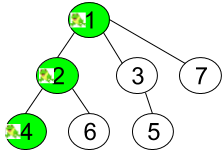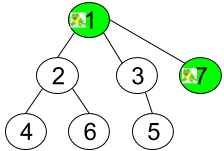# T 秒后青蛙的位置

## 1377. T 秒后青蛙的位置 (Hard)

• 在一秒内，青蛙从它所在的当前顶点跳到另一个 未访问 过的顶点（如果它们直接相连）。
• 青蛙无法跳回已经访问过的顶点。
• 如果青蛙可以跳到多个不同顶点，那么它跳到其中任意一个顶点上的机率都相同。
• 如果青蛙不能跳到任何未访问过的顶点上，那么它每次跳跃都会停留在原地。```输入：n = 7, edges = [[1,2],[1,3],[1,7],[2,4],[2,6],[3,5]], t = 2, target = 4

``````输入：n = 7, edges = [[1,2],[1,3],[1,7],[2,4],[2,6],[3,5]], t = 1, target = 7

```

```输入：n = 7, edges = [[1,2],[1,3],[1,7],[2,4],[2,6],[3,5]], t = 20, target = 6

```

• `1 <= n <= 100`
• `edges.length == n-1`
• `edges[i].length == 2`
• `1 <= edges[i], edges[i] <= n`
• `1 <= t <= 50`
• `1 <= target <= n`
• 与准确值误差在 `10^-5` 之内的结果将被判定为正确。

### 相关话题

[] [深度优先搜索] [广度优先搜索] []